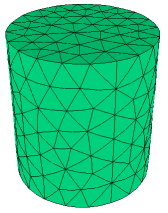﻿ Sample Problems > Usage > Eigenvalues > 3d_oildrum

# 3d_oildrum

Navigation:  Sample Problems > Usage > Eigenvalues >

# 3d_oildrum{ 3D_OILDRUM.PDE

*******************************************************************

This example illustrates the use of FlexPDE in Eigenvalue problems, or

Modal Analysis.

*******************************************************************

In this problem, we determine the four lowest-energy vibrational modes of

a circular cylinder, or "oil drum", clamped on the periphery.

What we see as results are the pressure distributions of the air inside the

drum.

The three-dimensional initial-boundary value problem associated with the

scalar wave equation for sound speed "c" can be written as

c^2*del2(u) - dtt(u) = 0,

with accompanying initial values and boundary conditions:

u = f(s,t)              on some part S1 of the boundary

dn(u) + a*u = g(s,t)    on the remainder S2 of the boundary.

If we assume that solutions have the form

u(x,y,z,t) = exp(i*w*t)*v(x,y,z)

(where "w" is a frequency) then the equation becomes

del2(v) + lambda*v = 0

with lambda = (w/c)^2, and with boundary conditions

v = 0                   on S1

dn(v) + a*v = 0         on S2.

The values of lambda for which this system has a non-trivial solution

are known as the eigenvalues of the system, and the corresponding solutions

are known as the eigenfunctions or vibration modes of the system.

}

title "Vibrational modes of an Oil Drum"

coordinates cartesian3

select

modes=4   { Define the number of vibrational modes desired.

The appearance of this selector tells FlexPDE

to perform an eigenvalue calculation, and to

define the name LAMBDA to represent the eigenvalues }

ngrid=6           { reduced mesh density for demo }

cell_limit = 3000   { keep problem small for demo }

 Variables     u   equations       {  the eigenvalue equation }    U: div(grad(u)) + lambda*u   = 0   { define the bounding z-surfaces } extrusion  z = -1,1       boundaries     { clamp the bottom and top faces }     surface 1 value(u) = 0       surface 2 value(u) = 0     { define circular sidewall }     Region 1                 start(0,-1)       value(u) = 0   { clamp the sides }       arc(center=0,0) angle 360   monitors             {  repeated for all modes }     contour(u) on x=0     contour(u) on y=0     contour(u) on z=1/2   plots                 {  repeated for all modes }     contour(u) on x=0   surface(u) on x=0     contour(u) on y=0   surface(u) on y=0     contour(u) on z=1/2   surface(u) on z=1/2   end